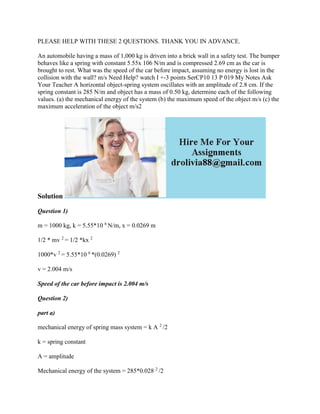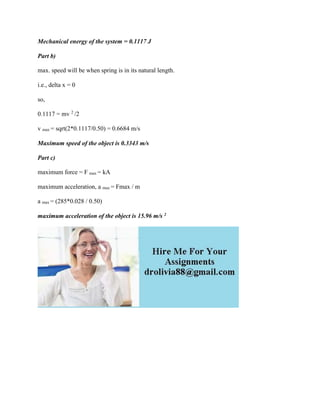Diese Präsentation wurde erfolgreich gemeldet.

Anzeige
Anzeige
Anzeige
Anzeige
Anzeige
Anzeige
Anzeige
Anzeige
Anzeige
Anzeige
Anzeige×

## Hier ansehen

1 von 2 Anzeige

An automobile having a mass of 1,000 kg is driven into a brick wall in a safety test. The bumper behaves like a spring with constant 5.55x 106 N/m and is compressed 2.69 cm as the car is brought to rest. What was the speed of the car before impact, assuming no energy is lost in the collision with the wall? m/s Need Help? watch I +-3 points SerCP10 13 P 019 My Notes Ask Your Teacher A horizontal object-spring system oscillates with an amplitude of 2.8 cm. If the spring constant is 285 N/m and object has a mass of 0.50 kg, determine each of the following values. (a) the mechanical energy of the system (b) the maximum speed of the object m/s (c) the maximum acceleration of the object m/s2
Solution
Question 1)
m = 1000 kg, k = 5.55*10 6 N/m, x = 0.0269 m
1/2 * mv 2 = 1/2 *kx 2
1000*v 2 = 5.55*10 6 *(0.0269) 2
v = 2.004 m/s
Speed of the car before impact is 2.004 m/s
Question 2)
part a)
mechanical energy of spring mass system = k A 2 /2
k = spring constant
A = amplitude
Mechanical energy of the system = 285*0.028 2 /2
Mechanical energy of the system = 0.1117 J
Part b)
max. speed will be when spring is in its natural length.
i.e., delta x = 0
so,
0.1117 = mv 2 /2
v max = sqrt(2*0.1117/0.50) = 0.6684 m/s
Maximum speed of the object is 0.3343 m/s
Part c)
maximum force = F max = kA
maximum acceleration, a max = Fmax / m
a max = (285*0.028 / 0.50)
maximum acceleration of the object is 15.96 m/s 2
.

An automobile having a mass of 1,000 kg is driven into a brick wall in a safety test. The bumper behaves like a spring with constant 5.55x 106 N/m and is compressed 2.69 cm as the car is brought to rest. What was the speed of the car before impact, assuming no energy is lost in the collision with the wall? m/s Need Help? watch I +-3 points SerCP10 13 P 019 My Notes Ask Your Teacher A horizontal object-spring system oscillates with an amplitude of 2.8 cm. If the spring constant is 285 N/m and object has a mass of 0.50 kg, determine each of the following values. (a) the mechanical energy of the system (b) the maximum speed of the object m/s (c) the maximum acceleration of the object m/s2
Solution
Question 1)
m = 1000 kg, k = 5.55*10 6 N/m, x = 0.0269 m
1/2 * mv 2 = 1/2 *kx 2
1000*v 2 = 5.55*10 6 *(0.0269) 2
v = 2.004 m/s
Speed of the car before impact is 2.004 m/s
Question 2)
part a)
mechanical energy of spring mass system = k A 2 /2
k = spring constant
A = amplitude
Mechanical energy of the system = 285*0.028 2 /2
Mechanical energy of the system = 0.1117 J
Part b)
max. speed will be when spring is in its natural length.
i.e., delta x = 0
so,
0.1117 = mv 2 /2
v max = sqrt(2*0.1117/0.50) = 0.6684 m/s
Maximum speed of the object is 0.3343 m/s
Part c)
maximum force = F max = kA
maximum acceleration, a max = Fmax / m
a max = (285*0.028 / 0.50)
maximum acceleration of the object is 15.96 m/s 2
.

Anzeige
Anzeige